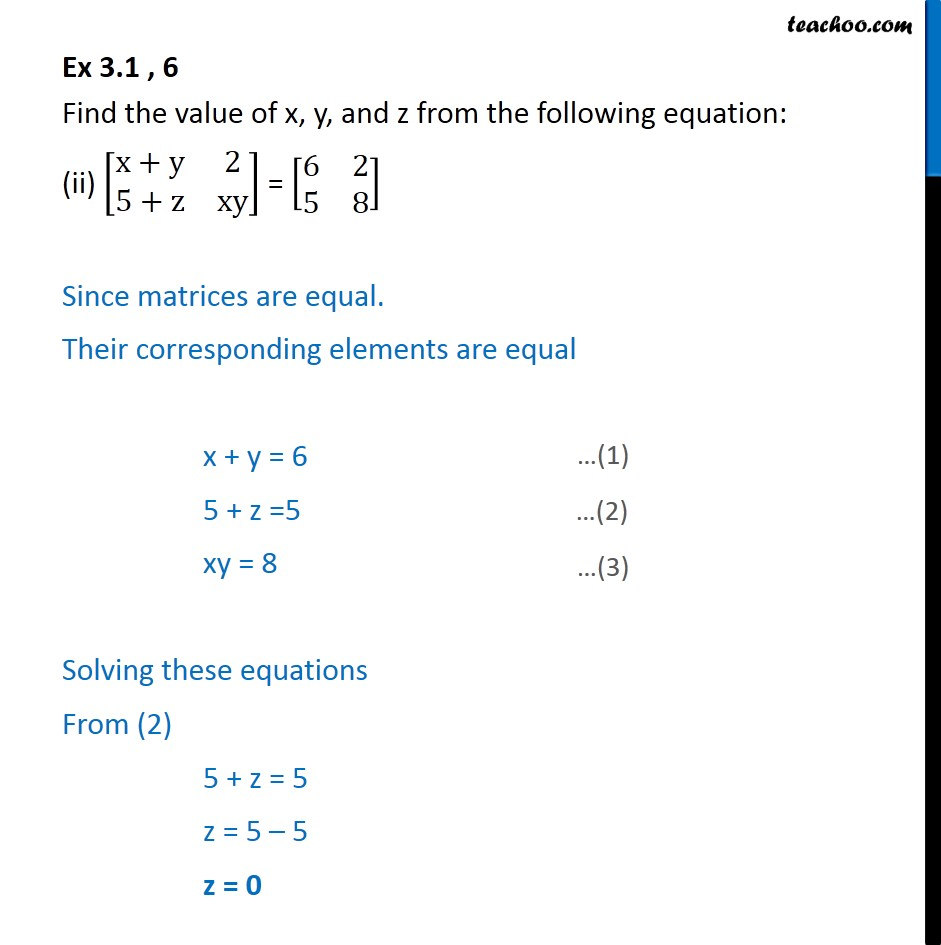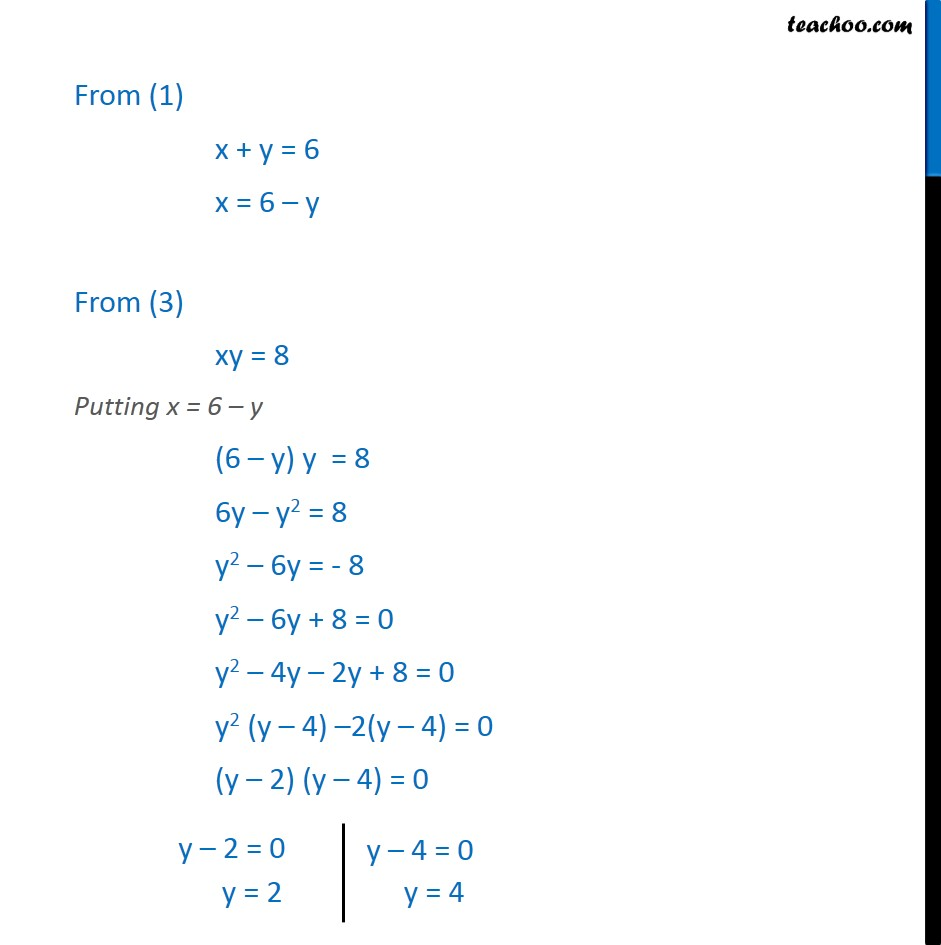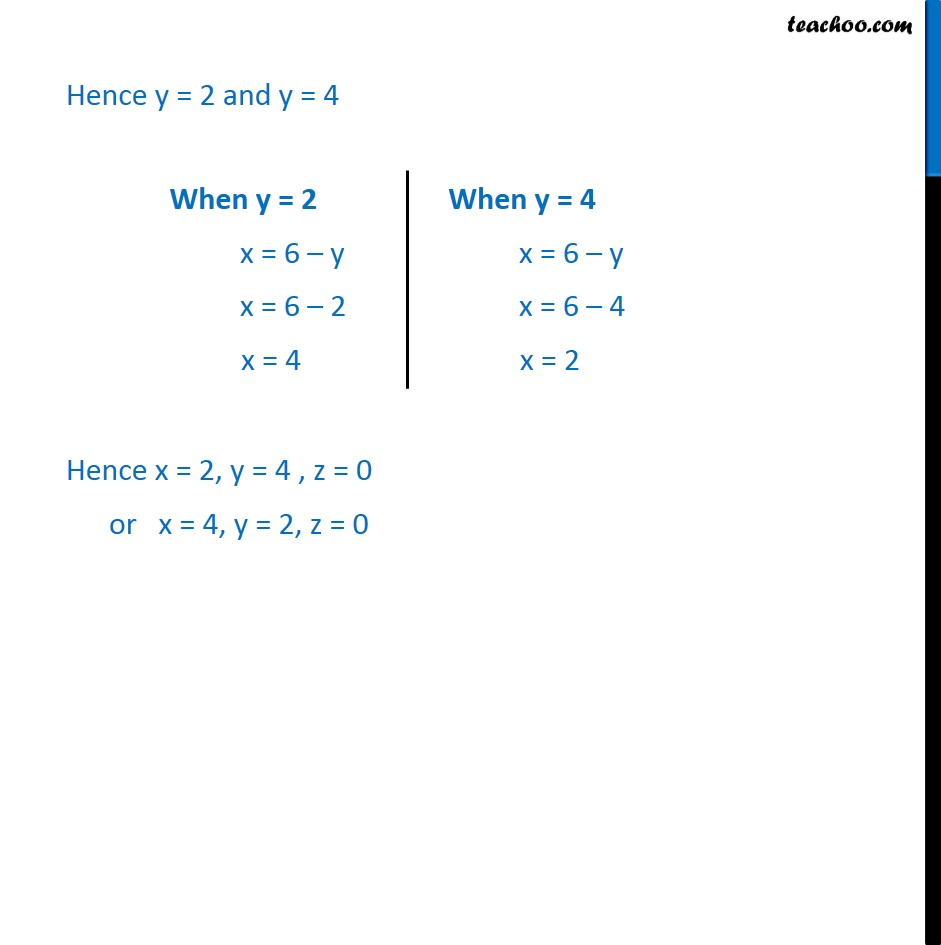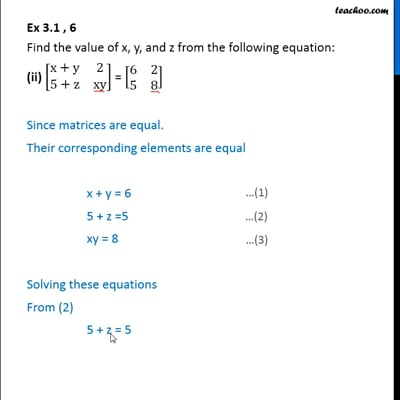Ex 3.1

Chapter 3 Class 12 Matrices
Serial order wiseThis video is only available for Teachoo black users

Introducing your new favourite teacher - Teachoo Black, at only ₹83 per month

### Transcript

Ex 3.1 , 6 Find the value of x, y, and z from the following equation: (ii) [ 8(x+y&2@5+z&xy)] = [ 8(6&2@5&8)] Since matrices are equal. Their corresponding elements are equal x + y = 6 5 + z =5 xy = 8 Solving these equations From (2) 5 + z = 5 z = 5 5 z = 0 From (1) x + y = 6 x = 6 y From (3) xy = 8 Putting x = 6 y (6 y) y = 8 6y y2 = 8 y2 6y = - 8 y2 6y + 8 = 0 y2 4y 2y + 8 = 0 y2 (y 4) 2(y 4) = 0 (y 2) (y 4) = 0 Hence y = 2 and y = 4 Hence x = 2, y = 4 , z = 0 or x = 4, y = 2, z = 0